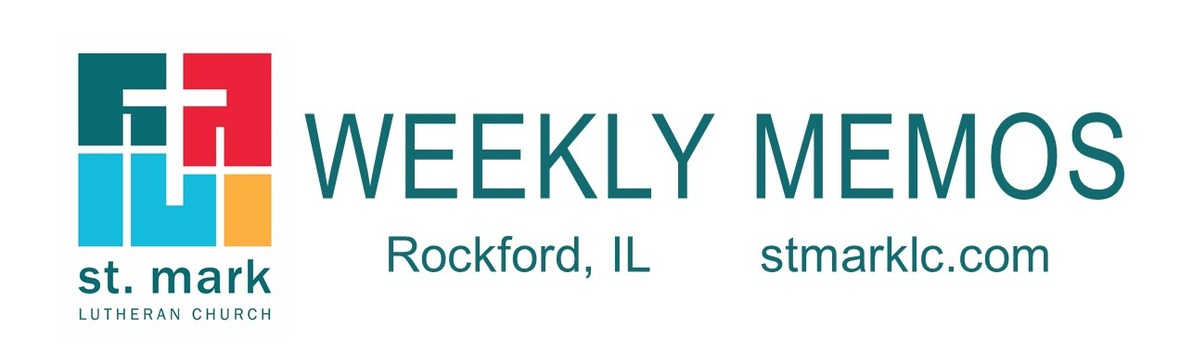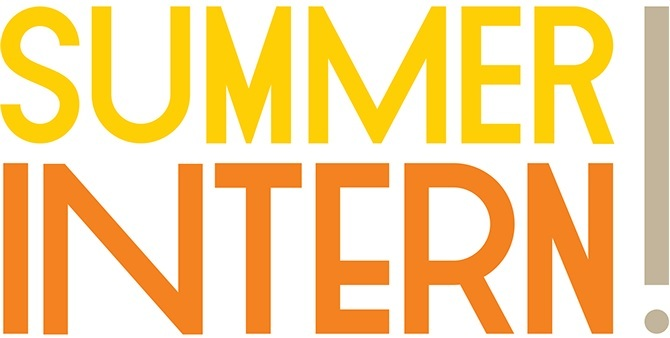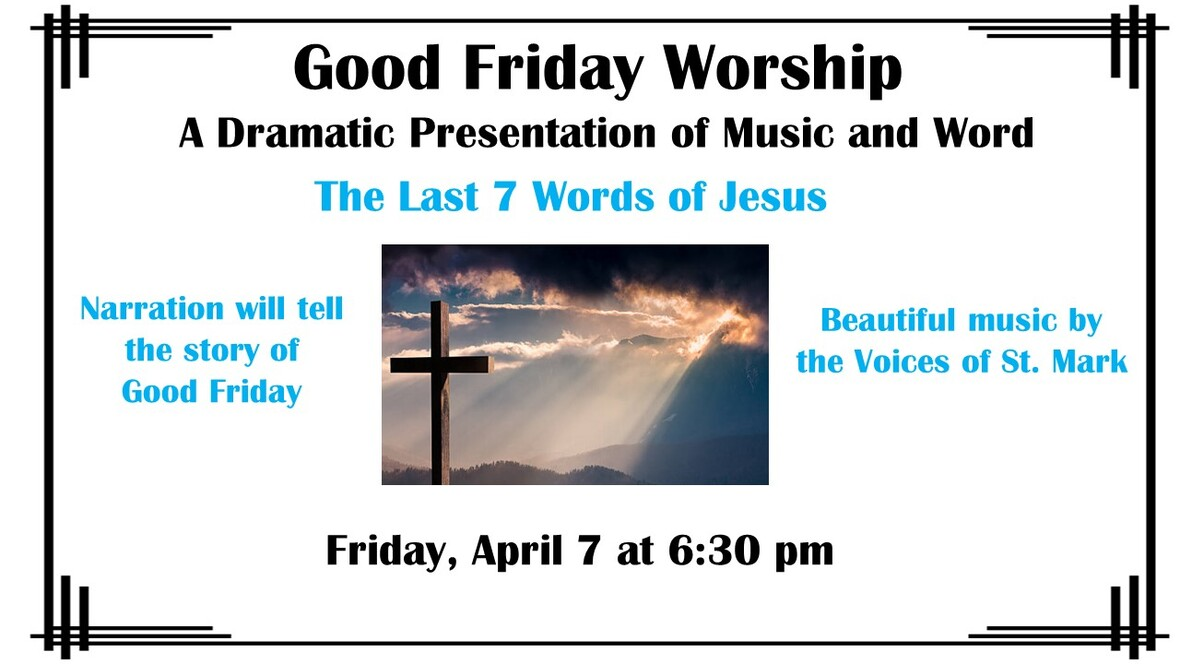table div table+table div table{width:100%;padding:0}table div table+table div table img{width:96.23%;padding:0;float:none}table div table+table div table td{width:100%;padding:0 1.88% 18px}/* styles */## This Week at St. Mark March 19-26, 2023

 table div table+table+table+table div table{width:100%;padding:0}table div table+table+table+table div table img{width:96.23%;padding:0;float:none}table div table+table+table+table div table td{width:100%;padding:0 1.88% 18px}/* styles */## Sunday, March 19

9:00 A.M. Worship with Live-Stream
9:00 A.M. Welcoming New Members in the Service
10:00 A.M. New Member Reception & Fellowship Hour with coffee and donuts
10:10 A.M. Sunday School
11:00 A.M. Worship in Fellowship Hall

## Monday, March 20

10:30 A.M. Classic Chorale Rehearses

## Tuesday, March 21

10:00 A.M. Staff Meeting
11:00 A.M. St. Mark Duplicate Bridge
7:00 P.M. Executive Meeting

## Wednesday, March 22 Lenten Worship Service

9:00 A.M. Women's Bible Study
9:00 A.M. Men's Bible Study
9:30 A.M. St. Mark Ringers
6:30 P.M. Wednesday Lenten Worship Service
7:15 P.M. Voices of St. Mark

## Thursday, March 23

9:00 A.M. Cherry Valley Bridge Group
12:30 P.M. Sew and Sews
6:30 P.M. Emotions Anonymous
7:15 P.M. Red Lights

## Friday, March 24

7:30 A.M. St. Mark Breakfast Group at Denny's Restaurant

## Saturday, March 25

6:00 P.M. Train Group

## Sunday, March 26

9:00 A.M. Worship with Live-Stream
9:00 A.M. Baptism of Noah Alex Fink
10:00 A.M. Fellowship Hour with coffee and donuts
10:10 A.M. Sunday School
11:00 A.M. Worship in Fellowship Hall
6:30 P.M. St. Mark Youth Group

 table div table+table+table+table+table+table div table{width:100%;padding:0}table div table+table+table+table+table+table div table img{width:96.23%;padding:0;float:none}table div table+table+table+table+table+table div table td{width:100%;padding:0 1.88% 18px}/* styles */## News and Events

 table div table+table+table+table+table+table+table+table div table{width:100%;padding:0}table div table+table+table+table+table+table+table+table div table img{width:96.23%;padding:0;float:none}table div table+table+table+table+table+table+table+table div table td{width:100%;padding:0 1.88% 18px}/* styles */table div table+table+table+table+table+table+table+table+table+table div table{width:100%;padding:0}table div table+table+table+table+table+table+table+table+table+table div table img{width:96.23%;padding:0;float:none}table div table+table+table+table+table+table+table+table+table+table div table td{width:100%;padding:0 1.88% 18px}/* styles */Welcome New Members
Sunday we will receive 15 new members (9 new families) to St. Mark. Mary and Ray Babineau, Jim Endres, Karen Endres, Alieta, Tim, Michael and Lucas Guntly, Len and Cathy Hoffmann, John Juliano, Nancy Lynch, Mary and Patrick Mallow and Roger Whitworth. Join us in Fellowship Hall with cake, coffee, and donuts as we welcome them to our church family.

 table div table+table+table+table+table+table+table+table+table+table+table+table div table{width:100%;padding:0}table div table+table+table+table+table+table+table+table+table+table+table+table div table img{width:96.23%;padding:0;float:none}table div table+table+table+table+table+table+table+table+table+table+table+table div table td{width:100%;padding:0 1.88% 18px}/* styles */Join us every Sunday in Lent as we explore By Heart: Conversations with Martin Luther’s Small Catechism, a multiple-week course designed to explore the biblical and historical context of the Small Catechism, its link to the church’s faith, and its connection to Luther’s life and your life today. Each session will consist of a short video, time for discussion, and an opportunity to hear the words of the Small Catechism in a new way.

 table div table+table+table+table+table+table+table+table+table+table+table+table+table+table div table{width:100%;padding:0}table div table+table+table+table+table+table+table+table+table+table+table+table+table+table div table img{width:96.23%;padding:0;float:none}table div table+table+table+table+table+table+table+table+table+table+table+table+table+table div table td{width:100%;padding:0 1.88% 18px}/* styles */Mid-Week Lenten Services
Starting Wednesday, March 1 at 6:30 pm we will gather to worship using Holden Evening Prayer, composed by Marty Haugen. Take a moment in the middle of your week to center yourself with this beloved service of candlelight and song. Due to the nature of this service, it will not be live-streamed. Join us every Wednesday at 6:30 pm in the Sanctuary during Lent.

 table div table+table+table+table+table+table+table+table+table+table+table+table+table+table+table+table div table{width:100%;padding:0}table div table+table+table+table+table+table+table+table+table+table+table+table+table+table+table+table div table img{width:96.23%;padding:0;float:none}table div table+table+table+table+table+table+table+table+table+table+table+table+table+table+table+table div table td{width:100%;padding:0 1.88% 18px}/* styles */Save the Date for VBS!
Mark your calendars: VBS is June 19-23!
Every day, our heroes will answer the call and spring into action, learning from Bible Heroes in stories from the Old and New Testaments to work together to bring peace, build each other up, and share with the whole world the great story of God’s love.

We will experience these stories together through music, storytelling, arts and crafts, games, and science. Come be part of our ultimate Hero Team!

Our Volunteer Team is assembling now – teens and adults are needed to help lead the Heroes through their adventures. Contact julie@stmarklc.com to volunteer! It will be the most fun week of your summer!

Registration, for kids who are completing PreK 4 – 5th grade, will open in May.

 table div table+table+table+table+table+table+table+table+table+table+table+table+table+table+table+table+table+table div table{width:100%;padding:0}table div table+table+table+table+table+table+table+table+table+table+table+table+table+table+table+table+table+table div table img{width:96.23%;padding:0;float:none}table div table+table+table+table+table+table+table+table+table+table+table+table+table+table+table+table+table+table div table td{width:100%;padding:0 1.88% 18px}/* styles */Easter Lilies
Each Easter we have our beautiful Sanctuary and Fellowship Hall decorated with Easter Lilies. It's that time to order yours. The price per plant is \$12.00. After the service, you may take your lily home or come to the church that week after Easter to pick yours up.

You may fill out an order form and pay at church on Sundays, or click here. The deadline to order is Tuesday, April 4.

 table div table+table+table+table+table+table+table+table+table+table+table+table+table+table+table+table+table+table+table+table div table{width:100%;padding:0}table div table+table+table+table+table+table+table+table+table+table+table+table+table+table+table+table+table+table+table+table div table img{width:96.23%;padding:0;float:none}table div table+table+table+table+table+table+table+table+table+table+table+table+table+table+table+table+table+table+table+table div table td{width:100%;padding:0 1.88% 18px}/* styles *//* styles */ Lenten Giving Project to Benefit MELD MELD, a program within the Youth Services Network, provides both emergency and transitional housing to youth and young parents. This year for Lent, Children’s Ministry and Outreach are hosting a donation drive to help them in this important work! MELD has specifically requested new or gently used children’s clothes in sizes 2T – 8, and activity supplies such as chalk, play-doh, bubbles, art supplies, coloring books, and card games. We will have bins at the entrance near the cafe and in Fellowship Hall. You can bring your items any time between now and Easter. If you would prefer to give a monetary gift, please write “MELD” in the memo line of your checks or on your cash envelope. You can also select the “MELD Lent Project” fund on PushPay. Your gifts will show the children at MELD shelters that they are loved and cared for by their community. Thank you so much!
 table div table+table+table+table+table+table+table+table+table+table+table+table+table+table+table+table+table+table+table+table+table+table div table{width:100%;padding:0}table div table+table+table+table+table+table+table+table+table+table+table+table+table+table+table+table+table+table+table+table+table+table div table img{width:96.23%;padding:0;float:none}table div table+table+table+table+table+table+table+table+table+table+table+table+table+table+table+table+table+table+table+table+table+table div table td{width:100%;padding:0 1.88% 18px}/* styles */Looking for a Summer Internship?
Attention College-aged members of St. Mark, the Summer Intern application for 2023 is live. Please click here at this link to fill out the application if you are interested in being the Summer Intern. If you have any questions please email Pastor
Katrina at prkatrina@stmarklc.com.

 table div table+table+table+table+table+table+table+table+table+table+table+table+table+table+table+table+table+table+table+table+table+table+table+table div table{width:100%;padding:0}table div table+table+table+table+table+table+table+table+table+table+table+table+table+table+table+table+table+table+table+table+table+table+table+table div table img{width:96.23%;padding:0;float:none}table div table+table+table+table+table+table+table+table+table+table+table+table+table+table+table+table+table+table+table+table+table+table+table+table div table td{width:100%;padding:0 1.88% 18px}/* styles */Holy Saturday Family Service
Relaxed, Multi-Sensory Worship for All Ages
April 8th, 4:00 pm

On Holy Saturday, the day before Easter, come experience what it means to find hope in an empty tomb. People of all ages are encouraged to come to this peaceful service, which will be unlike any other in Christianity’s most holy week. We will tell familiar Bible stories and journey with one another through worshipful moments that engage all our senses. A good portion of this service will be self-directed, which means you will be able to visit different areas in the sanctuary and worship at your own pace. Whether you come with others or by yourself, there will be plenty for you to discover about the great promise of Easter. Weather permitting, this service will begin outside before moving to the sanctuary. Accommodations will be made for those with mobility challenges.

 table div table+table+table+table+table+table+table+table+table+table+table+table+table+table+table+table+table+table+table+table+table+table+table+table+table+table div table{width:100%;padding:0}table div table+table+table+table+table+table+table+table+table+table+table+table+table+table+table+table+table+table+table+table+table+table+table+table+table+table div table img{width:96.23%;padding:0;float:none}table div table+table+table+table+table+table+table+table+table+table+table+table+table+table+table+table+table+table+table+table+table+table+table+table+table+table div table td{width:100%;padding:0 1.88% 18px}/* styles */Do you have the Church Directory App?
It's free and simple! It only takes a few minutes to download the app on your phone or create an online account. The Instant Church Directory App will always have updated members' information at your fingertips.

Mobiel App Login Instructions for Android Devices

For those of you who already have the app and you want to submit a new photo or if your contact information has changed, you can submit these changes from the app. Select Menu and Edit My Family. If you have any questions, please contact Jill Davenport at 815-398-3557 or jill@stmarklc.com.

 table div table+table+table+table+table+table+table+table+table+table+table+table+table+table+table+table+table+table+table+table+table+table+table+table+table+table+table+table div table{width:100%;padding:0}table div table+table+table+table+table+table+table+table+table+table+table+table+table+table+table+table+table+table+table+table+table+table+table+table+table+table+table+table div table img{width:96.23%;padding:0;float:none}table div table+table+table+table+table+table+table+table+table+table+table+table+table+table+table+table+table+table+table+table+table+table+table+table+table+table+table+table div table td{width:100%;padding:0 1.88% 18px}/* styles */The 2023 Altar Flower Chart is Up
Each Sunday we have two beautiful bouquets of flowers on the altar. The 2023 flower chart is up in the narthex on the bulletin board. You may sign up on the chart, call the church office or click on this link. We will continue to purchase them from Cherry Blossom. The price per arrangement will stay the same \$40. If you sign up online, put your memory or honor of in the comment line and pay here or write a check to St. Mark. If you have the mobile app you can do all of it on there. You may take your flowers home after the service or stop by the office
during the week to pick them up.

 table div table+table+table+table+table+table+table+table+table+table+table+table+table+table+table+table+table+table+table+table+table+table+table+table+table+table+table+table+table+table div table{width:100%;padding:0}table div table+table+table+table+table+table+table+table+table+table+table+table+table+table+table+table+table+table+table+table+table+table+table+table+table+table+table+table+table+table div table img{width:96.23%;padding:0;float:none}table div table+table+table+table+table+table+table+table+table+table+table+table+table+table+table+table+table+table+table+table+table+table+table+table+table+table+table+table+table+table div table td{width:100%;padding:0 1.88% 18px}/* styles */## Youth MinistryUPDATED Youth Group Schedule - Meets from 6:30-8:00 pm
March 19 - No St. Mark Youth Group
March 26 - St. Mark Youth Group
April 2 - No Youth Group - Palm Sunday
April 9 - No Youth Group - Easter Sunday
April 16 - 2nd Sunday Youth Group at St. Mark
April 23 - St. Mark Youth Group
April 30 - St. Mark Youth Group
May 7 - St. Mark Youth Group
May 14 - No Youth Group - Mother's Day
May 21 - 2nd Sunday Youth Group-Grace Lutheran
May 28 - No Youth Group - Memorial Day Weekend
June 11 - 2nd Sunday Youth Group at Volcano Falls

In June Youth Group will be on Wednesday evenings. Please watch for more information regarding our Summer Youth Group events.

 table div table+table+table+table+table+table+table+table+table+table+table+table+table+table+table+table+table+table+table+table+table+table+table+table+table+table+table+table+table+table+table+table+table div table{width:100%;padding:0}table div table+table+table+table+table+table+table+table+table+table+table+table+table+table+table+table+table+table+table+table+table+table+table+table+table+table+table+table+table+table+table+table+table div table img{width:96.23%;padding:0;float:none}table div table+table+table+table+table+table+table+table+table+table+table+table+table+table+table+table+table+table+table+table+table+table+table+table+table+table+table+table+table+table+table+table+table div table td{width:100%;padding:0 1.88% 18px}/* styles *//* styles */table div table+table+table+table+table+table+table+table+table+table+table+table+table+table+table+table+table+table+table+table+table+table+table+table+table+table+table+table+table+table+table+table+table+table+table+table div table{width:100%;padding:0}table div table+table+table+table+table+table+table+table+table+table+table+table+table+table+table+table+table+table+table+table+table+table+table+table+table+table+table+table+table+table+table+table+table+table+table+table div table img{width:96.23%;padding:0;float:none}table div table+table+table+table+table+table+table+table+table+table+table+table+table+table+table+table+table+table+table+table+table+table+table+table+table+table+table+table+table+table+table+table+table+table+table+table div table td{width:100%;padding:0 1.88% 18px}/* styles */table div table+table+table+table+table+table+table+table+table+table+table+table+table+table+table+table+table+table+table+table+table+table+table+table+table+table+table+table+table+table+table+table+table+table+table+table+table+table div table{width:100%;padding:0}table div table+table+table+table+table+table+table+table+table+table+table+table+table+table+table+table+table+table+table+table+table+table+table+table+table+table+table+table+table+table+table+table+table+table+table+table+table+table div table img{width:96.23%;padding:0;float:none}table div table+table+table+table+table+table+table+table+table+table+table+table+table+table+table+table+table+table+table+table+table+table+table+table+table+table+table+table+table+table+table+table+table+table+table+table+table+table div table td{width:100%;padding:0 1.88% 18px}/* styles */## Remember In Our Prayers

 table div table+table+table+table+table+table+table+table+table+table+table+table+table+table+table+table+table+table+table+table+table+table+table+table+table+table+table+table+table+table+table+table+table+table+table+table+table+table+table+table div table{width:100%;padding:0}table div table+table+table+table+table+table+table+table+table+table+table+table+table+table+table+table+table+table+table+table+table+table+table+table+table+table+table+table+table+table+table+table+table+table+table+table+table+table+table+table div table img{width:96.23%;padding:0;float:none}table div table+table+table+table+table+table+table+table+table+table+table+table+table+table+table+table+table+table+table+table+table+table+table+table+table+table+table+table+table+table+table+table+table+table+table+table+table+table+table+table div table td{width:100%;padding:0 1.88% 18px}/* styles */## Our St. Mark Members:

Mark Honzel, Kris Steinke, Elton and Sandy Olson, Lisa Belk, Lorine Kortus, Beth Johnson, Carol Kilts, Rita Olson, Fritzi Panjkovich, Bev Johnson, Jack Sowl, Jan Cotter, Diane Swanson, Sherri Lindquist, Bette Patterson.

## Our condolences to:

Vicki and Gary Foster upon the death of Gary's brother, Larry Foster.

## Family and Friends of St. Mark Members:

Terry Backen, Denise Nichols, Carrie Nack, Barbara, Buttchen, John Ayotte, Carrie Kielty, Ed Sobczyk, Elizabeth Woodworth, Carrie Nelson, Bill Hart, Ellie Krepfle, Tom Montgomery, Jon Baylander, John Mortensen, Carolyn Neagle, Jenny Hobby, Bill King, Carolyn King, Norma Comstock.

## Those serving in the military and their families:

Logan Gehrke, Hunter Haggestad, Tristan Davenport, Scot Hornick,
Bailey Panjkovich, Jim Tammen, Brandon Ballenger, Taylor Eversole.

Strengthen and encourage those in public health services and in the medical profession: care-givers, nurses, attendants, doctors, all who commit themselves to care for the sick and their families.

 table div table+table+table+table+table+table+table+table+table+table+table+table+table+table+table+table+table+table+table+table+table+table+table+table+table+table+table+table+table+table+table+table+table+table+table+table+table+table+table+table+table+table div table{width:100%;padding:0}table div table+table+table+table+table+table+table+table+table+table+table+table+table+table+table+table+table+table+table+table+table+table+table+table+table+table+table+table+table+table+table+table+table+table+table+table+table+table+table+table+table+table div table img{width:96.23%;padding:0;float:none}table div table+table+table+table+table+table+table+table+table+table+table+table+table+table+table+table+table+table+table+table+table+table+table+table+table+table+table+table+table+table+table+table+table+table+table+table+table+table+table+table+table+table div table td{width:100%;padding:0 1.88% 18px}/* styles *//* styles */ Office Hours Monday through Thursday 9:00 A.M. - 4:00 P.M. Friday - 9:00 A.M. - 1:00 P.M. Telephone: 815-398-3557 Publications St. Mark Memos, the weekly e-newsletter, is distributed each Thursday. The deadline for submission is Monday by 3:00 P.M. Please click to send submissions. Worship Services Miss a Sunday at St. Mark? Please visit our website and watch the service online. Please click to watch.
 table div table+table+table+table+table+table+table+table+table+table+table+table+table+table+table+table+table+table+table+table+table+table+table+table+table+table+table+table+table+table+table+table+table+table+table+table+table+table+table+table+table+table+table+table div table{width:100%;padding:0}table div table+table+table+table+table+table+table+table+table+table+table+table+table+table+table+table+table+table+table+table+table+table+table+table+table+table+table+table+table+table+table+table+table+table+table+table+table+table+table+table+table+table+table+table div table img{width:96.23%;padding:0;float:none}table div table+table+table+table+table+table+table+table+table+table+table+table+table+table+table+table+table+table+table+table+table+table+table+table+table+table+table+table+table+table+table+table+table+table+table+table+table+table+table+table+table+table+table+table div table td{width:100%;padding:0 1.88% 18px}/* styles */